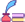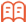##审稿期刊目次

## 期刊订阅

Open Access Article

Advances in International Applied Mathematics. 2022; 4: (1) ; 12-15 ; DOI: 10.12208/j.aam.20220002.

# Analysis of the Application of Mathematical Modeling in High School Mathematics数学建模在高中数学中的应用例析

*通讯作者： 贾明霞,单位：扬州大学数学科学学院 江苏扬州；

## 摘要

“数学建模”是数学六大核心素养之一，是运用数学知识解决实际问题的具体体现。本文通过举例介绍高中数学中几个重要数学模型（函数、不等式、数列、几何和概率统计模型）在高中数学中的应用。

## Abstract

“Mathematical modeling” is one of the six core competencies of mathematics, and it is the concrete embodiment of using mathematical knowledge to solve practical problems. This article introduces the application of several important mathematical models (functions, inequalities, sequence, geometry and probability and statistical models) in high school mathematics through examples.

Key words： High school mathematics; Mathematical modeling; Mathematical application

## 参考文献 References

 周锋.高中数学建模教学及学生数学应用能力的提高[J].数学学习与研究,2021（18）：135-136.

 罗志华.浅谈数学建模案例在高中数学教学中的应用举措[J].名师在线,2021（03）：8-9.

 吴榴红.数学建模思想在高中教学中的应用策略研究[J].中外交流,2021,28（3）：677.

 陆立强.对高中开展数学建模活动的若干思考和建议[J].数学建模及其应用,2019,8（03）：75-78.

 中华人民共和国教育部.普通高中数学课程标准（2017年版）[M].北京：人民教育出版社,2018．